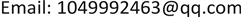﻿ 安徽省人口老龄化程度预测研究 Population Aging Degree Prediction Research in Anhui Province

Vol.07 No.08(2018), Article ID:26606,8 pages
10.12677/AAM.2018.78124

Population Aging Degree Prediction Research in Anhui Province

Yi Wang

College of Sciences, North China University of Technology, BeijingReceived: Aug. 3rd, 2018; accepted: Aug. 20th, 2018; published: Aug. 27th, 2018ABSTRACT

China has large population, the population issue has become an important reason for restricting China’s economic development. Anhui Province is the country’s most populated province, and in 1998, it entered the aging society. Due to the continued increase in the aging population, aging population has brought increasingly serious social, economic and other important problems. Therefore, the development of aging research and analysis without delay, to accurately predict the trend of population aging, and the stability of the socio-economic development of Anhui Province, is particularly important. This paper uses part of the data in1998~2015 in Anhui Province relating to the elderly population and the gray forecast GM(1,1) model prediction methods to predict the total population in Anhui Province and elderly population over the next decade, and then forecast the aging factor. The results showed that the total population in Anhui Province will continue to rise in next decade, and elderly population is also in the process of increasing, population aging coefficient subsequently continues to rise. Finally, according to the empirical model, the current situation and forecast results of Anhui population aging are analyzed and related policy recommendations are presented based on the results.

Keywords:Aging of Population, Grey Prediction, GM(1,1) Model1. 引言

2. 安徽省人口老龄化发展历程及成因

2.1. 安徽省人口老龄化发展历程

2.2. 安徽省人口老龄化成因

3. 预测方法的选择

3.1. 灰色预测法定义

3.2. 灰色预测模型的建立

${X}^{\left(1\right)}=\left\{{X}^{\left(1\right)}\left(1\right),{X}^{\left(1\right)}\left(2\right),\cdots ,{X}^{\left(1\right)}\left(n\right)\right\}$ (1)

$\frac{\text{d}{X}^{\left(1\right)}}{\text{d}t}+a{X}^{\left(1\right)}=\mu$ (2)

$\stackrel{^}{\alpha }$ 为待估参数向量， $\stackrel{^}{\alpha }=\left(\frac{a}{\mu }\right)$ ，可用最小二乘法求解，可得 $\stackrel{^}{\alpha }={\left({B}^{\text{T}}B\right)}^{-1}{B}^{\text{T}}{Y}_{n}$ ，其中，

$B=\left[\begin{array}{cc}-1/2\left[{X}^{\left(1\right)}\left(1\right)+{X}^{\left(1\right)}\left(2\right)\right]& 1\\ -1/2\left[{X}^{\left(1\right)}\left(2\right)+{X}^{\left(1\right)}\left(3\right)\right]& 1\\ ⋮& ⋮\\ -1/2\left[{X}^{\left(1\right)}\left(n-1\right)+{X}^{\left(1\right)}\left(n\right)\right]& 1\end{array}\right]$ , ${Y}_{n}=\left[\begin{array}{c}{X}^{\left(0\right)}\left(2\right)\\ {X}^{\left(0\right)}\left(3\right)\\ ⋮\\ {X}^{\left(0\right)}\left(N\right)\end{array}\right]$

${\stackrel{^}{X}}^{\left(1\right)}\left(k+1\right)=\left[{X}^{\left(0\right)}\left(1\right)-\frac{\mu }{a}\right]{\text{e}}^{-ak}+\frac{\mu }{a}$ ( $k=0,1,2,\cdots ,n$ )(3)

3.3. 模型的检验

${\Delta }^{\left(0\right)}\left(i\right)=|{X}^{\left(0\right)}\left(i\right)-{\stackrel{^}{X}}^{\left(0\right)}\left(i\right)|$ , $i=1,2,\cdots ,n$

$\varphi \left(i\right)=\frac{{\Delta }^{\left(0\right)}\left(i\right)}{{X}^{\left(0\right)}\left(i\right)}×100%$ , $i=1,2,\cdots ,n$

1) 计算原始序列的标准差： ${S}_{1}=\sqrt{\frac{\sum {\left[{X}^{\left(0\right)}\left(i\right)-{\overline{X}}^{\left(0\right)}\right]}^{2}}{n-1}}$

2) 计算绝对误差序列的标准差： ${S}_{2}=\sqrt{\frac{\sum {\left[{\Delta }^{\left(0\right)}\left(i\right)-{\overline{\Delta }}^{\left(0\right)}\right]}^{2}}{n-1}}$

3) 计算方差比： $C=\frac{{S}_{2}}{{S}_{1}}$

4) 计算小误差概率： $P=P\left\{|{\Delta }^{\left(0\right)}\left(i\right)-{\overline{\Delta }}^{\left(0\right)}|<0.6745{S}_{1}\right\}$

${e}_{i}=|{\Delta }^{\left(0\right)}\left(i\right)-{\overline{\Delta }}^{\left(0\right)}|$${S}_{0}=0.6745{S}_{1}$ ，则 $P=P\left\{{e}_{i}<{S}_{0}\right\}$

4. 模型建立、预测结果及分析

4.1. 样本选取和数据说明Table 1. Accuracy inspection level reference tableTable 2. Population ageing in Anhui Province from 1998 to 2015

4.2. 安徽省人口总数以及老年人口数GM(1,1)模型建立

$\begin{array}{l}{x}^{\left(0\right)}=\left(6152,6205,6278,6325,6369,6410,6461,6516,6593,6676,\\ \text{\hspace{0.17em}}\text{\hspace{0.17em}}\text{\hspace{0.17em}}\text{\hspace{0.17em}}\text{\hspace{0.17em}}\text{\hspace{0.17em}}\text{\hspace{0.17em}}\text{\hspace{0.17em}}\text{\hspace{0.17em}}\text{\hspace{0.17em}}6741,6795,6827,6876,6902,6929,6936,6949\right)\end{array}$

${\stackrel{^}{x}}^{\left(1\right)}\left(k+1\right)=829032{\text{e}}^{0.0075k}+822880$ (4)Table 3. Results of total population forecast for Anhui Province from 1998 to 2015

$\begin{array}{l}{x}^{\left(0\right)}=\left(431.87,457.93,476.50,512.33,541.37,589.08,600.87,656.81,669.85,\\ \text{\hspace{0.17em}}\text{\hspace{0.17em}}\text{\hspace{0.17em}}\text{\hspace{0.17em}}\text{\hspace{0.17em}}\text{\hspace{0.17em}}\text{\hspace{0.17em}}\text{\hspace{0.17em}}\text{\hspace{0.17em}}715.67,751.62,776.67,698.40,784.55,833.76,848.11,812.21,815.12\right)\end{array}$

${\stackrel{^}{x}}^{\left(1\right)}\left(k+1\right)=14162.37{\text{e}}^{0.0351k}+13730.5$ (5)

4.3. 预测结果与分析Table 4. Results of the elderly population in Anhui Province from 1998 to 2015Table 5. Forecast results of population aging in Anhui Province from 2016 to 2025

5. 结论与相关政策建议

Population Aging Degree Prediction Research in Anhui Province[J]. 应用数学进展, 2018, 07(08): 1063-1070. https://doi.org/10.12677/AAM.2018.78124

1. 1. 杜鹏, 邬沧萍. 人口老龄化与老龄问题[M]. 北京: 中国人口出版社, 2006.

2. 2. 徐国祥. 统计预测和决策[M]. 上海: 上海财经大学出版社, 2012.

3. 3. 陈光慧, 蔡远飞, 李凤. 我国人口老龄化趋势预测与结构分析——基于非参数自回归模型[J]. 西北人口, 2014(4): 81-87.

4. 4. 陈毅华, 李永胜, 苏昌贵, 等. 径向基神经网络模型在人口老龄化预测中的应用——以湖南省为例[J]. 经济地理, 2012, 32(4): 32-37.

5. 5. 陈婷, 徐丽丽, 陈晓静, 等. 中国人口老龄化问题的预测与分析[C]//中国优选法统筹法与经济数学研究会. 第十三届中国管理科学学术年会论文集. 北京: 中国优选法统筹法与经济数学研究会, 2011: 711-716.

6. 6. 徐超然. 贵州省人口老龄化灰色关联分析与预测[J]. 现代经济信息, 2013(18): 491.

7. 7. 余倩, 吕盛鸽. 浙江省人口老龄化预测分析[J]. 杭州电子科技大学学报(社会学版), 2008(3): 24-28.